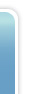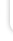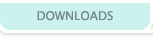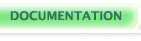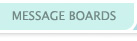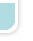## Fixed-point Integers

### An overview of fixed-point notation

Fixed-point is a way of treating whole numbers as both whole and fractional parts. It's called fixed point because you decide how much precision you need. Do you need to be able to represent halves? Tenths? Hundredths? Thousandths?

You can sort of think of fixed-point as a "secret code" for numbering. I'm sure you've heard of such numbering systems as binary, hexadecimal, and octal. Binary uses only 1s and 0s. Octal uses the numbers 0 through 7. Hexadecimal uses the numbers 0 through 9 and also the letters A through F.

Fixed-point also has a special sytstem for representing numbers. One example could be the number 100. You could decide, for example, that you want your fixed-point system to be able to track precision into the tenths. In that case, you could say that every ten numbers represent 1 whole number. The number 100, then, would end up being representative of the number 10.

How is that pulled off? The numbers 0 through 9 would equate to 0, 10 through 19 would equal 1, 20 through 29 would equal 2, etc. You can basically "decode" a system like this by dividing a number by 10. This division yields the integer part of your number. The fractional part is the number modulus 10. If you are unfamiliar with modulus, it's just the remainder of a division. For example, 13/10 equals 1 with a remainder of 3, so 3 is your fractional part.

When dealing with tenths, you're always going to have a fractional part of 0 through 9. In this case, these values correspond directly to .0, .1, .2, etc. However, if you decide to go with different precisions, this will not always be the case. If you were dealing in fourths for example (division and modulus by 4), you would have to take that into account when interpreting the fractional part. Fractionl parts are basically percentages of a whole number, so with fourths the fractional part is going to be one of 0, 1, 2, or 3. 0 would be 0% or .0, 1 would be 1/4th or .25, 2 would be 2/4ths or .5, and 3 would be 3/4ths or .75.

You may see a lot of >>8 or >>16 going on in fixed-point examples in C all over the web. This is basically the same as dividing by 256 or 65,536. Usually you'll see >>8 when dealing with 16-bit numbers, since 8 is half that many bits, or >>16 when dealing with 32-bit numbers for the same reason. These systems are capable of precision up to 256ths or 65,536ths, basically. Sometimes people aren't too picky about how much precision is needed, which is why they'll just split things down the middle like that. Bit shifting can also be faster, which is another reason you'll see it all over the place. As long as you understand the concept, you can do it any which-way you want!

See Also: Book of Hook - An Introduction to Fixed Point Math
Talkback

Post a new comment?

Talkback #1 written by zaril on 2004-09-18.

'If you are unfamiliar with modulus, it's just the remainder of a division. For example, 13/10 equals 1 with a remainder of 3, so 3 is your fractional part.'

To make it a bit clearer. To say modulus is a remainder of a division can be quite confusing since the remainder of the division 13/10 could easily be seen as 0.3 and not 3 which is the remainder of the modulus 13%10. Modulus could be one of these two explanations:

1. A type of division that returns; the fraction of the quotient times the divisor. 13 / 10 = 1.3; now the fraction of the quotient is .3 which we multiply by the divisor: .3 * 10 = 3; Since Verge doesn't handle fractions (which is why we're talking fixed-point) you should use fixed-point.

2. A subtraction that ocurrs continously as long as the divisor in a modulus is smaller or the same as the dividend. Technically it might not work this way, however the results would be the same, which makes it a theoretically ok way of seeing it.

35%10 would make the computer peel off 10 from 35 until you get a number smaller than 10. In this case you'd get 5.

Post a new comment?

 Doc Nav The VERGE 3 Manual Variables & Types in VC Integers Fixed-point Integers Strings Arrays Structs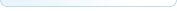Your docs View All Docs If you log in, you can edit the documentation, or create your own documents and tutorials!Ben McGraw's lovingly crafted this website from scratch for years.
It's a lot prettier this go around because of Jon Wofford.
Verge-rpg.com is a member of the lunarnet irc network, and would like to take this opportunity to remind you that regardless how babies taste, it is wrong to eat them.